INDIRECT function

The INDIRECT function returns the reference specified by a text string.

The INDIRECT function syntax is as follows:

= INDIRECT ( reference_text ; reference_style )

Reference text can be any text that the function can “convert” to a cell reference.

Reference style represents the style of referencing cells in Excel. If omitted or TRUE, reference text is interpreted as an A1 style reference i.e. the default style of referencing cells in Excel. If FALSE, reference text is interpreted as an R1C1 style reference. Unless you for whatever reason specifically want to use R1C1 style references, feel free to omit the reference style argument and use the shortened syntax:

= INDIRECT ( reference_text )

Consider the following example: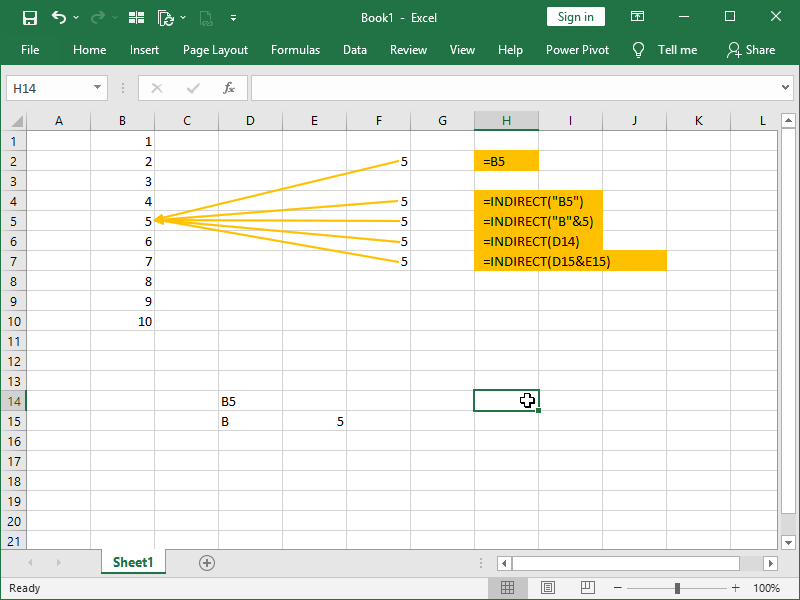In the column B, we have a series of values.

In the column F, we have a series of formulas. All of those formulas are referring to the B5 cell, i.e., they are all returning the value from the B5 cell.

Multiple ways of writing INDIRECT formula in the cells F4:F7 make no difference, basic principle is the same – we are writing and/or generating text that could be a valid cell reference, and the INDIRECT formula is converting that text to a cell reference.

While that is valid, it is not obviously useful. It does become so, however, if we combine INDIRECT function with other functions: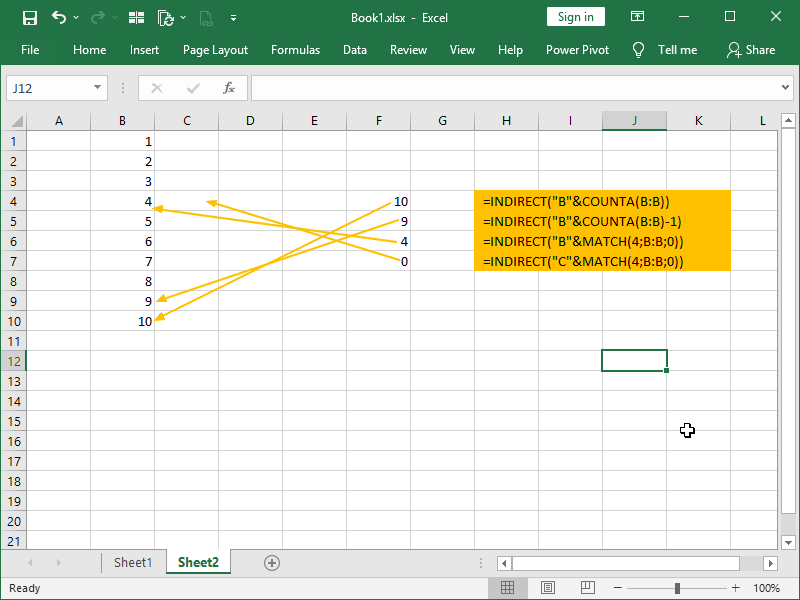We see that with the help of the COUNTA function we can count the non-empty cells in the column B. Assuming that there are no blanks, we can always easily return the value from the last row, or from the row before the last row.

We can also MATCH any given value in our column. If there is a match, we will return row number of the first match to the INDIRECT formula – in this case we are using INDIRECT MATCH combination in the same way we would use INDEX MATCH combination.

In principle, we can use any function to generate a text that could be a valid cell reference, both column and row part of the address, and the INDIRECT formula to convert that text to a cell reference.

We can also use the INDIRECT function to generate links to different sheets and workbooks.

Consider the following example: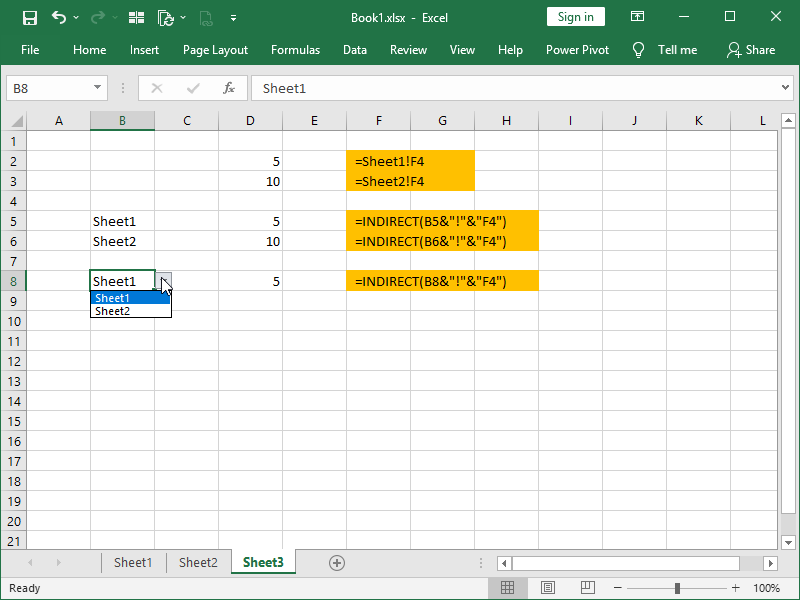We can return the value form the Sheet1 cell F4 by directly linking to it.

If we want, we can also refer to it by writing text “Sheet1!F4” and converting that text to reference with the help of the INDIRECT function.

However, if we create an input cell, such as cell B8 in this example, we can refer to that input cell in our INDIRECT formulas, and change our data based on our inputs.

We can do the same with Excel workbooks we are linking to: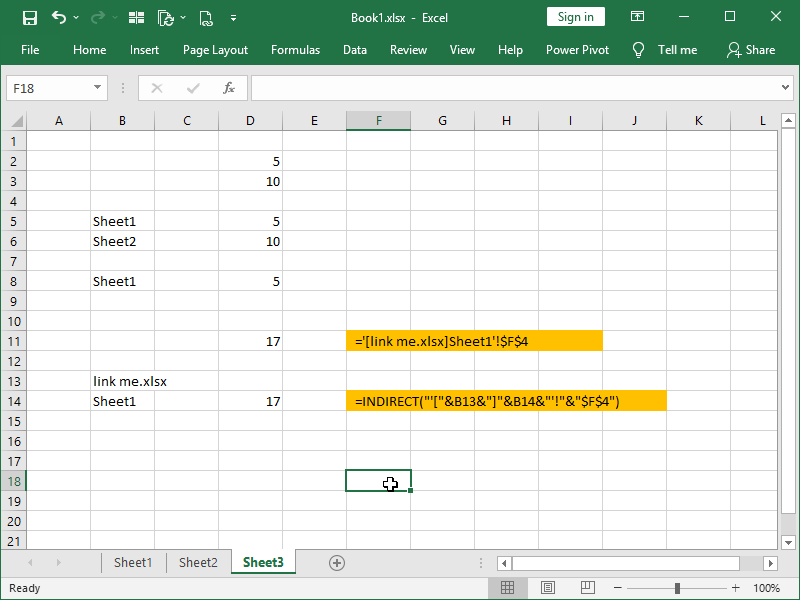In the cell D11 we are linking directly to the workbook “link me.xlsx”, but in the cell D14 we are again using inputs form cells B13 and B14 and generating a cell address located in the workbook “link me.xlsx”.

A few notes:

• #REF! error will be returned if our text to be converted to a reference is not a valid reference,
• in order for our reference to be valid, we will have to include all the needed special characters such as exclamation mark[!], apostrophe [‘] and even square brackets [] in our text string,
• we can not use the INDIRECT function in order to refer to a closed workbook. Even with a valid address, combinations such as =INDIRECT(“‘C:\files\[link me.xlsx]Sheet1’!\$F\$4”) will also return a #REF! error.

Another thing to note is that deleting or inserting rows and columns does not affect the references in the INDIRECT function. Remember, inside of the parentheses of the INDIRECT function is text converted to reference when the function is calculated. For example, if we insert a new cell F4 in Sheet2 and we shift cells down, the following will happen: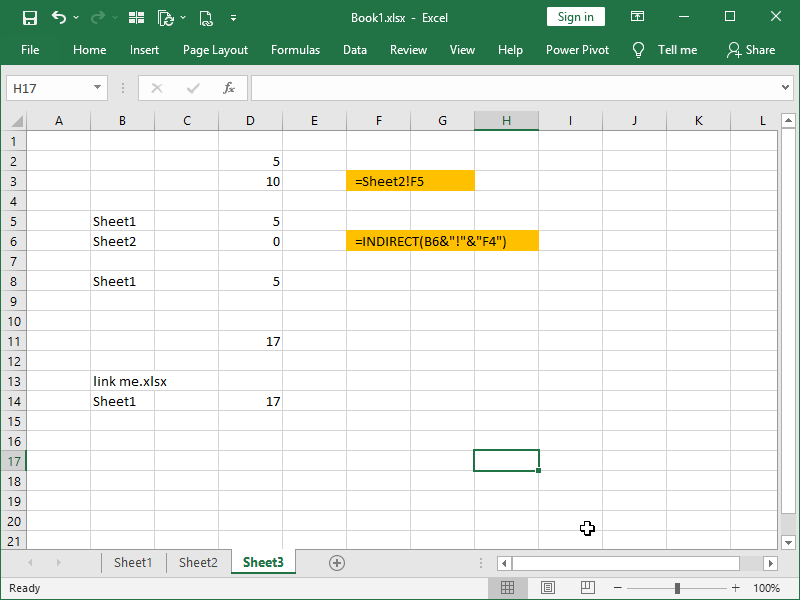D1 cell formula is now referring to cell Sheet2!F5, while D6 cell formula is still referring to (now empty) cell Sheet2!F4, and will continue to do so!

This kind of behavior is sometimes desired when dealing with cell ranges.

Assume that you want to always, no matter what, SUM the first ten rows of a given column – the INDIRECT function is the easiest solution for that job.

We can see in the following example how the INDIRECT function behaves when cell ranges are reference text: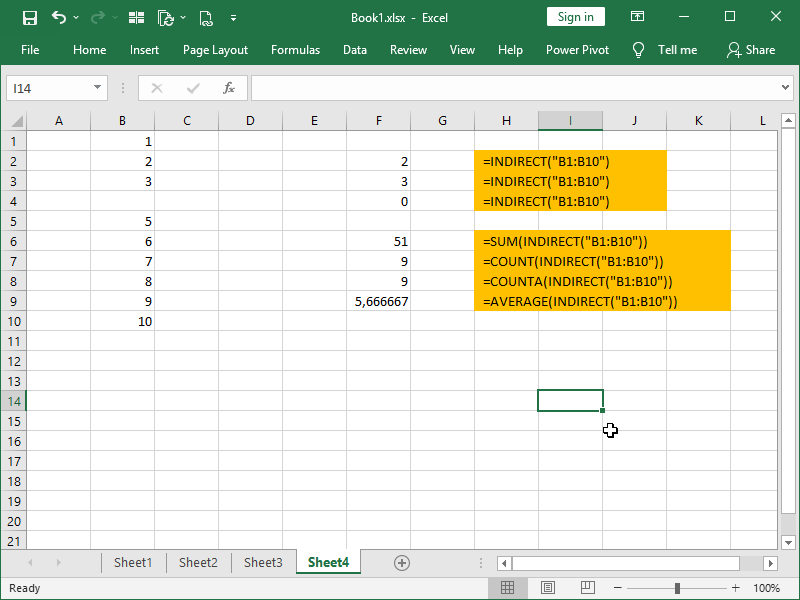Returning cell ranges with the INDIRECT function makes sense only if we do something with that return!

Dig deeper:

Text in Excel